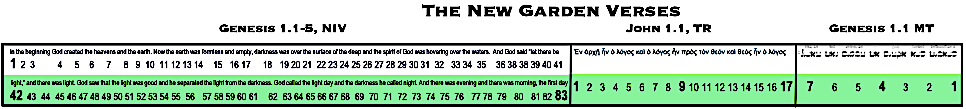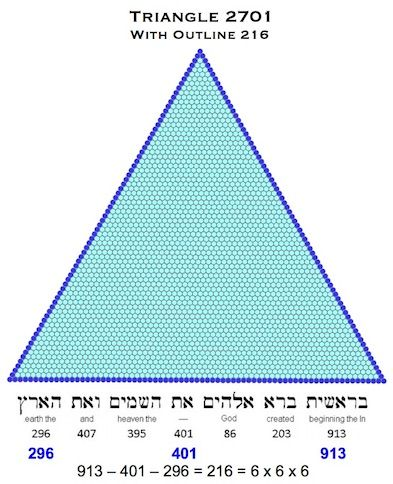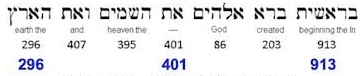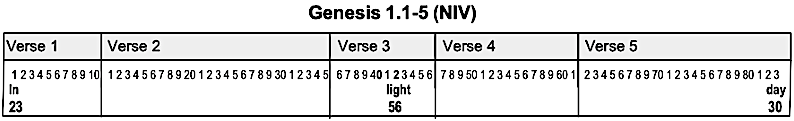# Signed in TriplicateIntroduction

In the New Bible Code, the Hebrew and Greek codes and in Jewish and Christian tradition, the number three has always symbolised completion (beginning, middle, end) and divine perfection. The Cube, extending equally into the three spatial dimensions, is the symbol of perfection in Judaism. In Christanity, the Trinity is the source and ultimate symbol of the perfection of three. The triangle with three vertices is a potent symbol of the Trinity and both the triangle and the cube have been extensively employed by the Creator in these codes, an unbreakable threefold cord of word, number and form.

The New Garden of Eden is itself a complete, divinely-perfect, three part seal, comprising of three portions of text from three manuscripts in three languages. These verses were meant to be joined, as witnessed by the geometry and the alphanumeric identities they combine to create and which populate the New Garden. Accordingly, the New Garden's final authentication comes from a three-part signature that firmly locks these verses together and simultaneously reveals the identity of the Gardener Himself.

The New Garden

Here is the three part seal I call the New Garden once more.The three-part signature is found by the simple procedure of summing, the initial, central and final words in each part of the seal, emboldened above. This technique has been employed before in these codes, an early example being the encoding of the outline of triangle 2701, which is the sum of the words in the Hebrew Genesis 1.1 (BHS), within the first, central and final words of the verse.So let's look at each portion of text and highlight the initial, central and final words, as I did above.

1. Genesis 1.1 (BHS)Hebrew is read right to left, so the first word, Bereshith (In the beginning), sums to 913, the middle word, aleph-tav (untranslatable), sums to 401 and the final word, ha eretz (and the earth), sums to 296.

2. John 1.1 (TR)So the first word, En (In), sums to 55, the middle word, en (was), sums to 58 and the final word, logos (word), sums to 373

3. Genesis 1.1-5 (NIV)There are too many words to show them all, but the first word, 'In', sums to 23, the central word, 'light', sums to 56, and the final word, 'day', sums to 30.

The Three Sums

If we now sum the values for the initial, central and final word triplets, we obtain the following totals:

 Sum of First Word Values Sum of Central Word Values Sum of Final Word Values 913 + 55 + 23              = 991 401 + 58 + 56 = 515 296 + 373 + 30 = 699 Jesus the Messiah (s) = 991 Jesus (s)         = 515 Lord Jesus (s)   = 699

So all three sums are fundamental numerical values for Jesus Christ!

When we subtract the central value, 515, from the values either side (991 and 699), we glimpse two more sparkling facets of this finely-cut gem.

 991 - 515            .= 476 699 - 515   = 184 The Messiah (s)  = 476 Lord (s)      = 184

If we now take the sum of sums we find this piece of code is marked with the overall theme of this site:

 991 + 515 + 699 = 2205 = 441 x 5 Appearing (c) = 441

Finally, if we sum the positional values of each of these words, we have the following supporting identities:

 Sum of initial word positions Sum of middle word positions Sum of final word positions 1 + 1 + 1        = 3 4 + 9 + 42                   = 55 7 + 17 + 83         = 107 Divine perfection Jesus the Messiah (r) = 55 The Messiah (o) = 107

Again we have Jesus the Messiah, these supporting identities self-consciously reflecting the gematria-based encodings. The total sum here is 165, or 55 x 3, multiplying Jesus the Messiah with the divine perfection of 3 to point to the miracle that gave rise to both Christianity and the code itself.

Resurrection (o) = 165

Summary

This neat little piece of code completely validates the three-part seal that is the New Garden of Eden and assures us in these uncertain times that the rest of the information planted in the Garden and indeed within all three Biblical texts is genuine, supremely important and, as these numerical signatures witness, comes to us directly from the Creator Himself. Perhaps more importantly, the precision of these codes assures us that the the Bible itself, perhaps uniquely among literary works, can be trusted down to the last jot and tittle.

Bill Downie 16/11/22